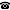# 2014 seminar talk: Dropping Polishness

Talk held by Andrea Medini (KGRC) at the KGRC seminar on 2014-05-22.

### Abstract

Classical descriptive set theory studies the subsets of complexity $\mathbf{\Gamma}$ of a Polish space $X$, where $\mathbf{\Gamma}$ is one of the (boldface) Borel or projective pointclasses. However, the definition of a $\mathbf{\Gamma}$ subset of $X$ extends in a natural way to spaces $X$ that are separable metrizable, but not necessarily Polish.

When one “drops Polishness”, many classical results suggest new problems in this context. We will discuss some early examples, then focus on the perfect set property. More precisely, we will determine the status of the statement “For every separable metrizable $X$, if every $\mathbf{\Gamma}$ subset of $X$ has the perfect set property then every $\mathbf{\Gamma}'$ subset of $X$ has the perfect set property” as $\mathbf{\Gamma},\mathbf{\Gamma}'$ range over all pointclasses of complexity at most analytic.

Kurt Gödel Research Center for Mathematical Logic. Währinger Straße 25, 1090 Wien, Austria.+43-1-4277-50501. Last updated: 2010-12-16, 04:37.